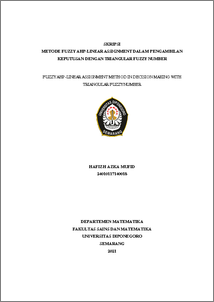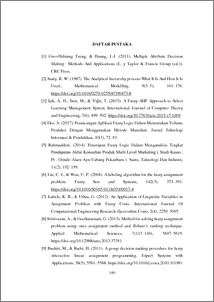# FUZZY AHP-LINEAR ASSIGNMENT METHOD IN DECISION MAKING WITH TRIANGULAR FUZZY NUMBER

Mufid, Hafizh Azka (2022) FUZZY AHP-LINEAR ASSIGNMENT METHOD IN DECISION MAKING WITH TRIANGULAR FUZZY NUMBER. Undergraduate thesis, UNDIP.Preview
PDF
274KbPDF
Restricted to Repository staff only

1632KbPreview
PDF
180Kb

## Abstract

Various events have different problems so that there is a need for a decision-making process (MCDM) in determining the policies. There are various methods for MCDM problems, one of that is the Analytical hierarchy process which is a decision-making process by describing multi-criteria problems that create a structured and systematic hierarchy. Fuzzy AHP-linear assignment combines three methods, fuzzy logic to solve the problem of selecting and analyzing hierarchical structures, the AHP method to obtain the weight of the criteria in decision making and the linear assignment method to obtain optimal ranking. The purpose is to determine the process of MCDM and its implementation so that the results of the FAHP - linear assignment calculation with F-AHP are compared. By using the FAHP linear assignment method, the ranking of UD BAS employees performance and evaluation will be determined by processing UD BAS employee appraisal data. The results are A A A A A 2 1 3 5 4     with the best alternative A2 and the best assignment P1 . Meanwhile, the F-AHP method results are A A A A A 2 4 5 3 1     with its ‘alternative A2 . The ranking results are slightly different because the ranking method in F-AHP is taking the highest criterion weight so that based on the number of criteria weights for each alternative while the FAHP-linear assignment method can reduce subjectivity by directly calculating the relative approach of each alternative for the optimal solution and providing a general preference rating of the alternatives for the criteria so as to provide an optimal assignment for each alternative. However, calculations using both methods give the same result. Keywords : Decision making, employee performance appraisal, fuzzy AHP, linear – assignment, ranking. Triangular fuzzy number

Item Type: Thesis (Undergraduate) Q Science > QA Mathematics Faculty of Science and Mathematics > Department of Mathematics 84303 INVALID USER 14 Jun 2022 10:13 14 Jun 2022 10:13

Repository Staff Only: item control page